# RSoft Application Gallery Note: Modes of a Slot Waveguide Structure

## Tool Used: FemSIM

This example uses FEM (finite element method) to analyze a slot waveguide structure . In exploring the computation of the fundamental TE mode of this structure using FemSIM, we will have to explore the convergence of the modes based on the mesh size for tightly confined structures. We will also look at the use of a non-uniform mesh to speed up the calculation time.

## Structure Overview

This device has two silicon channel waveguides that are buried in silica. A large discontinuity exists on the boundaries of the silicon waveguides due to the high index contrast. The waveguides are placed with a small gap between them, which causes the modes and discontinuities to overlap leading to a high confinement. The structure is composed of two identical buried Si channels in a uniform SiO2 background and separated by a small distance. The index values at a wavelength of 1.55 μm are: n1 = 3.48 and n2 = 1.44.

## Calculating the Fundamental TE Mode with Coarse Grid

FemSIM solves for the modes of the structure on a mesh. Since this structure is symmetric, we can speed up the computation by using a half-domain. The resulting mesh is shown below where the symmetric boundary is at x=0, and the x>=0 portion of the domain is simulated. The computed mode profile is also shown.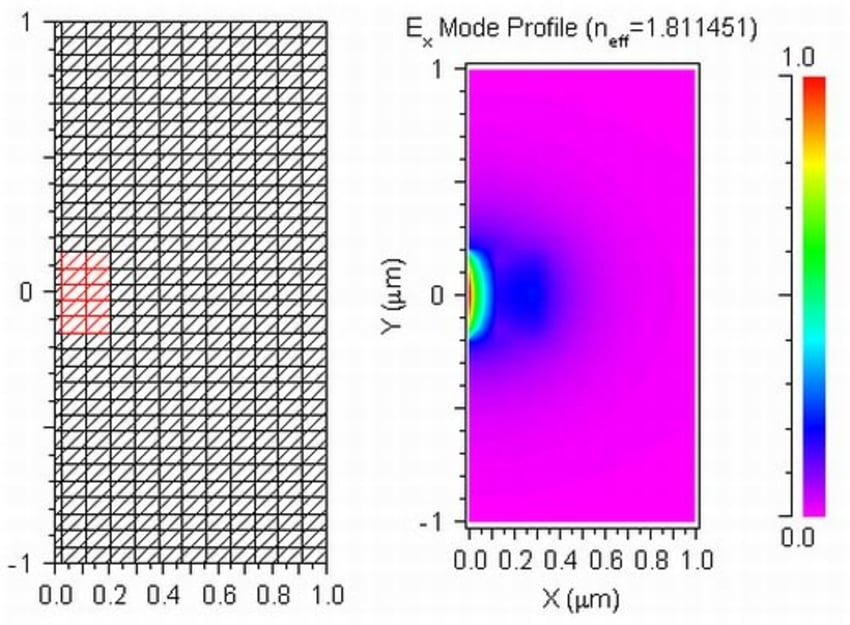Note that half the domain is shown in these plots.

## Convergence Study

Since we used a relatively coarse grid size, it will be beneficial to perform a convergence study on the grid size. Our convergence study will consist of continually reducing the grid size along X until the results converge to a particular result. To do this, we decrease the mesh spacing as width/NGX, where width =0.18 μm, and NGX has the following values: 5, 10, and 25.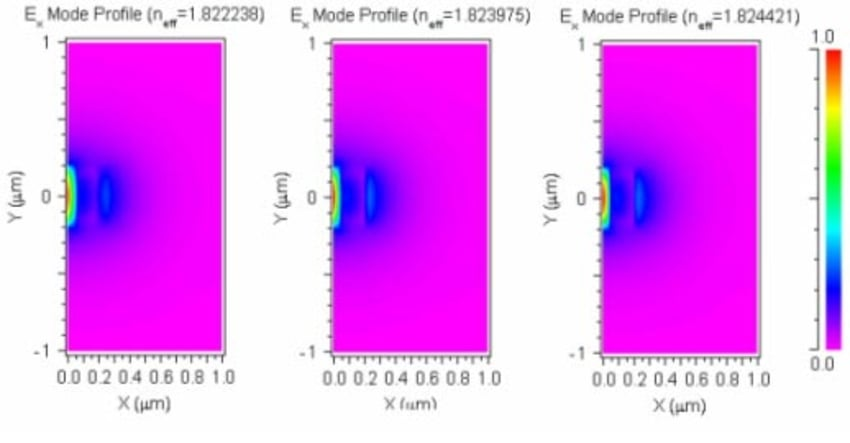As you can see, the effective index values results are converging as we increase the value of NGX. Also, as we increase NGX, the simulation time and memory also increase. For NGX = 25 the cross section of the mode at Y=0 is: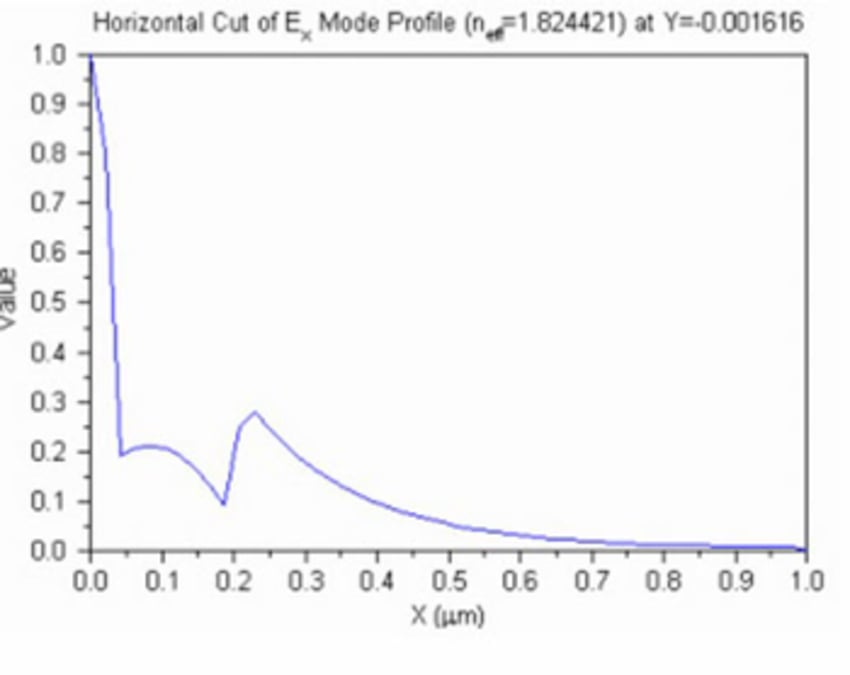The results are clearly beginning to get close to convergence. However, since the gap width is much smaller than the other feature sizes, it will take a lot of mesh points to converge to a solution. Rather than continue to reduce the mesh sizes further, we will utilize the advanced non-uniform grid options.

## Convergence Study Using Advanced Grid Features

The simulations that were previously run used a "global" grid size that affected the entire domain. However, as we can clearly see by the field plots shown above, a lot of interesting effects occur on the boundaries between the core and the cladding. FemSIM has the ability to use a non-uniform grid at interfaces between material regions, which allows for finer resolution in these areas without having to increase the resolution in the entire domain. Using only NGX=2 plus non-uniform refinement at the material boundaries (0.0015μm), we obtain the following mesh and mode profile: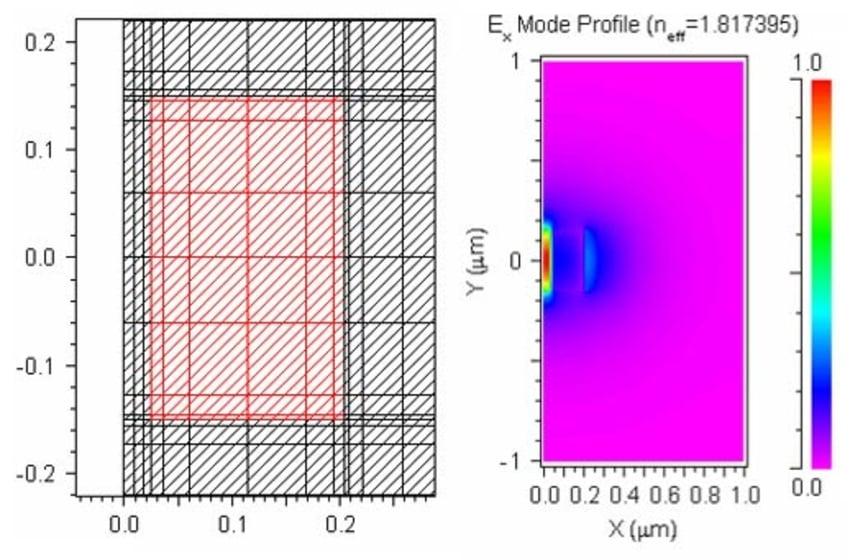The computations using the non-uniform grid options not only run relatively quicker than the previous simulations, but they result in a higher resolution field around the two core regions: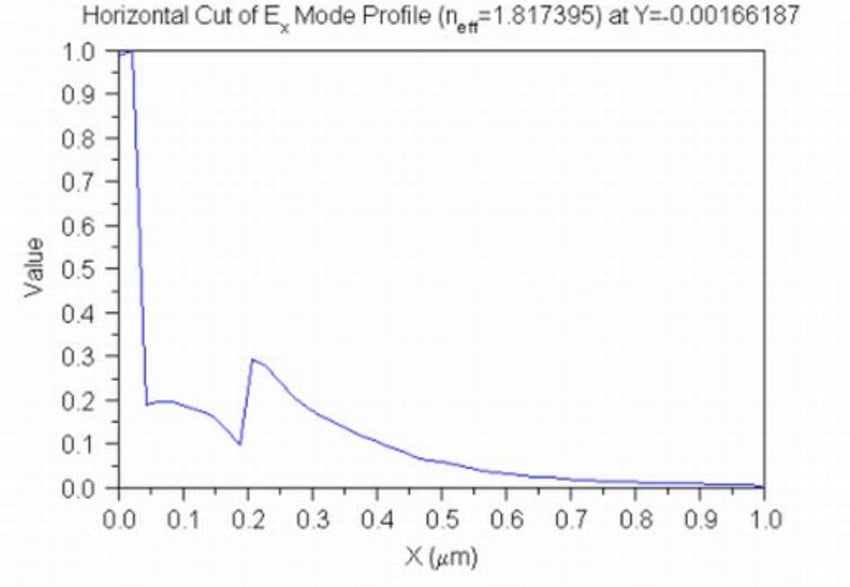The cross-section above matches Figure 2 of reference . By using these advanced parameters, we were able to compute the mode of this device with improved accuracy without changing the simulation speed appreciably.

## References:

 V. Almeida, Q. Xu, C. Barrios, and M. Lipson, "Guiding and confining light in a void nanostructure," Optics Letters, 29 (2004) 1209-1211.